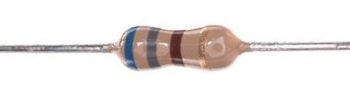calculator.org

# property>electrical resistance

## What is Electrical Resistance?The electrical resistance is a measure of the resistive force to the flow of an electric current. The resistance of a component with uniform cross-section will be proportional to length and the resistivity of the material. It will also be in inverse proportion to the cross-sectional area.

The discovery of electrical resistance was made by Georg Ohm in the late 1820s. The SI unit, the ohm, is named after him, and has the symbol Ω (capital Greek letter omega). The reciprocal quantity is electrical conductance which is measured in siemens (sometimes the slightly fanciful term 'mho' is used).

We can find out the value of a resistance by Ohm's Law, as it determines the amount of current passing through the object in relation to the potential difference across the object:

V = I.R, I = V/R or R = V/I

where I is the current passing through the object (in amperes), V is the potential difference across the object (Volts) and R is the resistance (measured in ohms). For most material and conditions the resistance is constant with respect to the amount of current or voltage, although in many cases it is dependent on temperature. These equations assume direct current (DC) and that the component being measured is a passive component.

An Ohm Meter is an instrument that can measure the resistance. A simple ohm meter is a constant voltage source (possibly nothing more than a battery) in series with an ammeter. Common ohm meters cannot easily measure low resistance very accurately as the resistance of the measuring leads cause significant voltage drop which interferes with the measurement. For very high resistances a correspondingly high voltage is required for accurate measurement.

DC resistance is the resistance R of a conductor and can be calculated as:-

R = (L . ρ) / A

where L is the length of the conductor, A is the cross-sectional area, and ρ (Greek letter rho) is the resistivity of the material. The equation provides good accuracy for long thin conductors such as wires. However, if the wire is conducting high frequency AC then the effective cross-sectional area will be reduced because of the skin effect. This can be mitigated by bundling many smaller conductors together.

Bookmark this page in your browser using Ctrl and d or using one of these services: (opens in new window)